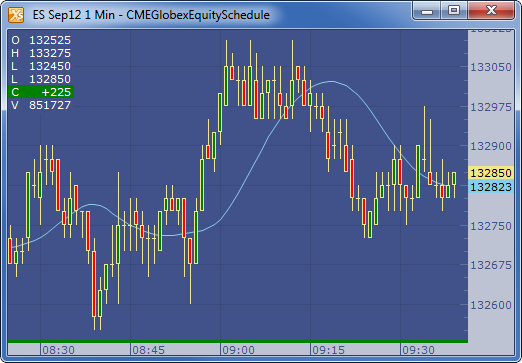← Back to X_TRADER® Help Library

### X_STUDY® Documentation

#### T3 (T3)

##### Description

The Triple Exponential Moving Average (T3) by Tim Tillson attempts to offers a moving average with better smoothing then traditional exponential moving average.

##### Formula

The Triple Exponential Moving Average (T3) of time series 't' is:

EMA1 = EMA(x,Period)

EMA2 = EMA(EMA1,Period)

GD = EMA1*(1+vFactor)) - (EMA2*vFactor)

T3 = GD (GD ( GD(t, Period, vFactor), Period, vFactor), Period, vFactor);

Where vFactor is a volume factor between 0 and 1 which determines how the moving averages responds. A value of 0 returns an EMA. A value of 1 returns DEMA. Tim Tillson advised or preferred a value of 0.7.

##### Example